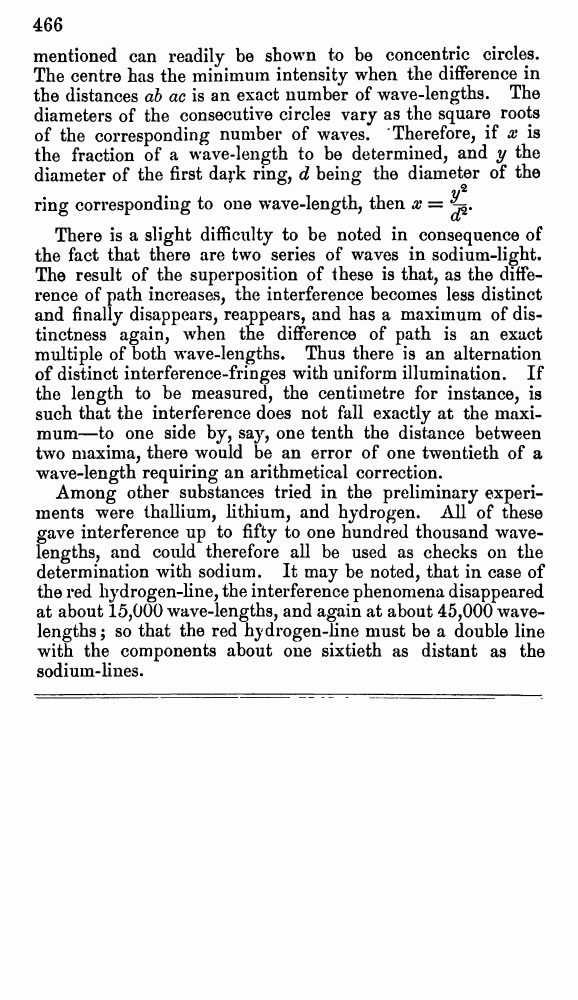# A. Michelson and E. Morley. On the Relative Motion of the Earth and the Luminiferous Æther. // Phil. Mag. S. 5. Vol. 24. No. 151. Dec. 1887.

В начало   Другие форматы   <<<     Страница 466   >>>449  450  451  452  453  454  455  456  457  458  459  460  461  462  463  464  465  466 mentioned can readily be shown to be concentric circles. The centre has the minimum intensity when the difference in the distances ab ac is an exact number of wave-lengths. The diameters of the consecutive circles vary as the square roots of the corresponding number of waves. Therefore, if x is the fraction of a wave-length to be determined, and y the diameter of the first dark ring, d being the diameter of the ring corresponding to one wave-length, then There is a slight difficulty to be noted in consequence of the fact that there are two series of waves in sodium-light. The result of the superposition of these is that, as the difference of path increases, the interference becomes less distinct and finally disappears, reappears, and has a maximum of distinctness again, when the difference of path is an exact multiple of both wave-lengths. Thus there is an alternation of distinct interference-fringes with uniform illumination. If the length to be measured, the centimetre for instance, is such that the interference does not fall exactly at the maximum—to one side by, say, one tenth the distance between two maxima, there would be an error of one twentieth of a wave-length requiring an arithmetical correction. Among other substances tried in the preliminary experiments were thallium, lithium, and hydrogen. All of these gave interference up to fifty to one hundred thousand wavelengths, and could therefore all be used as checks on the determination with sodium. It may be noted, that in case of the red hydrogen-line, the interference phenomena disappeared at about 15,000 wave-lengths, and again at about 45,000 wavelengths; so that the red hydrogen-line must be a double line with the components about one sixtieth as distant as the sodium-lines.